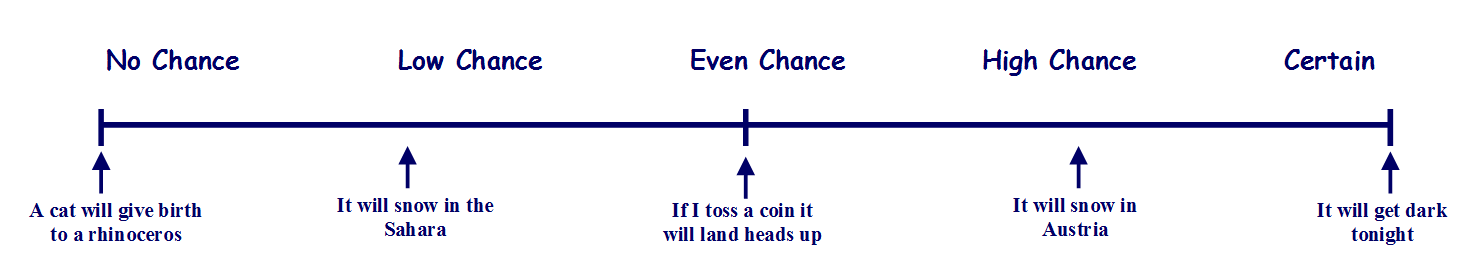# Handling Data - Probability

### Probability can also be defined in mathematical terms, as fractions, decimals and percentages.

_________________________________________________

### To begin with lets concentrate on understanding the vocabulary.

If we know that something will definitely happen, like "It will get dark tonight" then we are certain. If something is likely to happen but not definite, like "It will snow in Austria" then we can say there is a 'good chance ' or there is a 'high probability'. If something is unlikely to happen but not impossible, like "It will snow in the Sahara" then we can say there is a 'poor chance' or 'low probability'. If something cannot happen, if it is impossible, like "A cat will give birth to a rhinoceros" then we can say that there is 'no chance'.

What if the likelihood of something happening is the same as it not happening, like "If I toss a coin it will land heads up" we can say that it is 'equally likely' or has an 'equal or even chance' or it has a 'fifty-fifty chance'.

Use these worksheets from Mathsblog to practice using this vocabulary.
Probability 1                 Probability 2

Probability can be represented as a line called a Probability Scale.Use these worksheets from Mathsblog to practice using Probability Scales.
Probability 3                 Probability 4

_________________________________________________

### Now lets look at probability defined in mathematical terms, as fractions, decimals and percentages.

We need to think of how many outcomes are possible of an event occurring.

If you toss a coin, there are only two possible outcomes - heads or tails, it must be one or the other. So one thing will happen out of two possible outcomes -
1 out of 2 which represented as a fraction is ½.

To convert this to a decimal we divide the numerator by the denominator - 1 ÷ 2 = 0.5

and to convert to a percentage we multiply the decimal by 100 ... 0.5 x 100 = 50%

So the probability of a coin landing heads up is ½ or 0.5 or 50% (50% is where the term fifty-fifty comes from)

What if something is certain to happen, like 'It will get dark tonight'. There is only one possible outcome, it will get dark. So one thing will happen out of one possible outcome.
1 out of 1 ... 1/1 as a fraction (1) or 1 as a decimal or 1 x 100 = 100%

If there is no chance of something happening then there are no possible outcomes, so the probability is zero, 0 .... as a percentage 0 x 100 = 0%

What is the probability of choosing a red marble out of a bag containing 1 red,
1 blue, 1 yellow and 1 green marble?

There are four marbles in the bag so there are four possible outcomes - you could pick a red marble, a blue marble, a yellow marble or a green marble. So one thing will happen out of four possible outcomes. 1 out of 4

1/4 as a fraction, 1 ÷ 4 = 0.25 as a decimal and 0.25 x 100 = 25% as a percentage.

What is the probability of rolling a standard dice and getting an even number?

In this case there are six possible outcomes - 1, 2, 3, 4, 5, or 6, but three out of those six outcomes are even, 2, 4 and 6.      3 out of 6

As a fraction 3/6 or simplified 1/2 ... as a a decimal 1 ÷ 2 = 0.5 ... as a percentage 50%

Use these worksheets from Mathsblog to practice working out probability as a fraction,
decimal and percentage.

Probability 5
Probability 6

And these Probability and Chance

worksheet from Primary Resources

Probability Worksheet from BBC Schools